Manipulating the ideal gas equation PV =nRT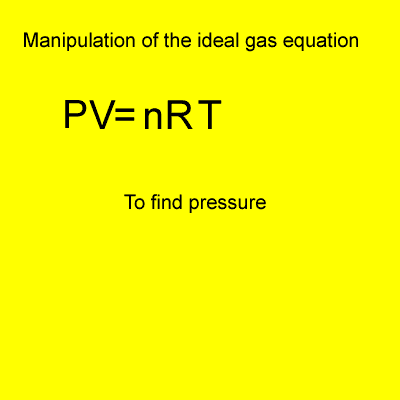n = mole d = density (g/L) M = molar mass of the gas under investigation P = pressure (kPa) T = temperature(K) V = volume(litres) R = 8.31J/K/mol The best way to tackle the ideal gas equation is to get straight into using it. Click on a formula in the column on the right to try several exercises using that particular formula.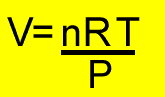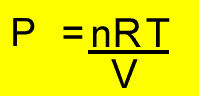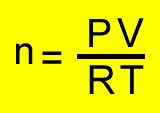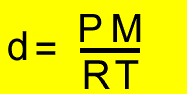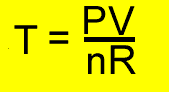Home